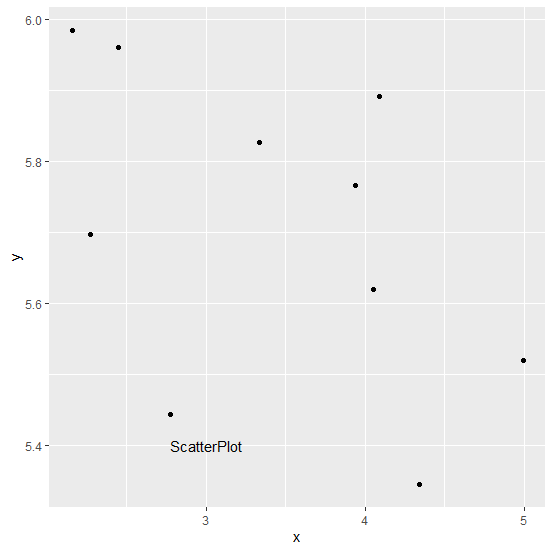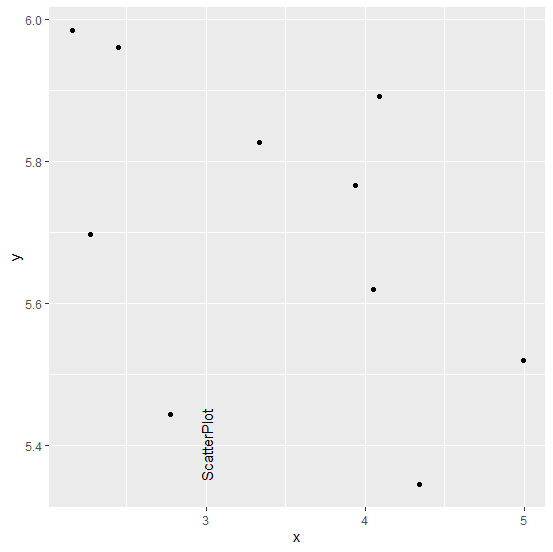# How to change the angle of annotated text in plot created by using ggplot2 in R?

To annotate the text inside a plot created by ggplot2, we can use annotate function. It is used to give some explanation about the plot or add any useful information that will help readers to understand the plot in a better way. Sometimes, we might want to change the angle of the annotated text, especially in cases where we have some information that is presented vertically in the plot, therefore, we can use angle argument of the annotate function.

## Example

Consider the below data frame −

Live Demo

> x<-runif(10,2,5)
> y<-runif(10,5,6)
> df<-data.frame(x,y)
> df

## Output

      x       y
1 4.086537 5.890591
2 2.271184 5.697052
3 3.335322 5.827102
4 2.155897 5.984699
5 4.054110 5.620492
6 3.936053 5.766108
7 4.341102 5.345369
8 2.450337 5.960743
9 4.992243 5.520061
10 2.776401 5.443892

> library(ggplot2)
> ggplot(df,aes(x,y))+geom_point()+annotate("text",x=3,y=5.4,label="ScatterPlot")

## OutputChanging the angle of text “ScatterPlot” −

> ggplot(df,aes(x,y))+geom_point()+annotate("text",x=3,y=5.4,label="ScatterPlot",angle=9
0)

## Output